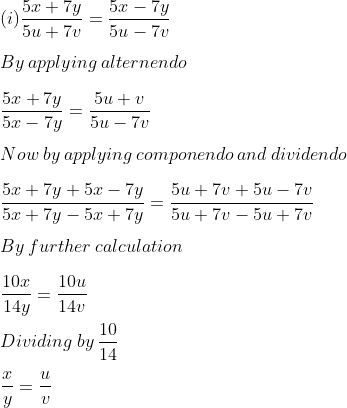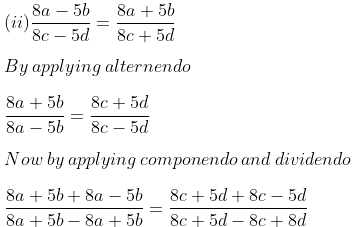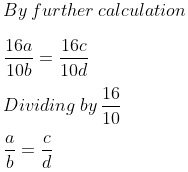Guru

# (i) If 5x+7y/5u+7v=5x−7y/5u−7v , Show that x/y=u/v (ii) 8a−5b/8c−5d=8a+5b/8c+5d , prove that a/b=c/d

• 0

This is an important ques from the Book ML Aggarwal class 10th,, chapter – 7, ratio and proportion.

It has been asked in previous year question paper of 2008

In this ques we have been given that

(i) 5x+7y/5u+7v=5x−7y/5u−7v , and we have to apply mathematical operations to prove that x/y=u/v and

(ii) 8a−5b/8c−5d=8a+5b/8c+5d ,and we ahve6to prove that a/b=c/d

Question no. 2, 7.3

Share

1. Solution:Therefore, it is proved.Therefore, it is proved.

• 0